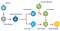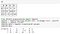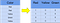# Data Preprocessing in Python

## An implementation

At the heart of Machine Learning is to process data. Your machine learning tools are as good as the quality of your data. This blog deals with the various steps of cleaning data. Your data needs to go through a few steps before it is could be used for making predictions.Machine Learning process diagram

The Dataset for this blog can be accessed from here.

# Steps involved in data preprocessing :

1. Importing the required Libraries
2. Importing the data set
3. Handling the Missing Data.
4. Encoding Categorical Data.
5. Splitting the data set into test set and training set.
6. Feature Scaling.

So let us look at these steps one by one.

## Step 1: Importing the required Libraries

To follow along you will need to download this dataset : Data.csv

Every time we make a new model, we will require to import Numpy and Pandas. Numpy is a Library which contains Mathematical functions and is used for scientific computing while Pandas is used to import and manage the data sets.

Here we are importing the pandas and Numpy library and assigning a shortcut “pd” and “np” respectively.

## Step 2: Importing the Dataset

Data sets are available in .csv format. A CSV file stores tabular data in plain text. Each line of the file is a data record. We use the read_csv method of the pandas library to read a local CSV file as a dataframe.

After carefully inspecting our dataset, we are going to create a matrix of features in our dataset (X) and create a dependent vector (Y) with their respective observations. To read the columns, we will use iloc of pandas (used to fix the indexes for selection) which takes two parameters — [row selection, column selection].

## Step 3: Handling the Missing DataAn example of Missing data and Imputation

The data we get is rarely homogenous. Sometimes data can be missing and it needs to be handled so that it does not reduce the performance of our machine learning model.

To do this we need to replace the missing data by the Mean or Median of the entire column. For this we will be using the sklearn.preprocessing Library which contains a class called Imputer which will help us in taking care of our missing data.

Our object name is imputer. The Imputer class can take parameters like :

1. missing_values : It is the placeholder for the missing values. All occurrences of missing_values will be imputed. We can give it an integer or “NaN” for it to find missing values.
2. strategy : It is the imputation strategy — If “mean”, then replace missing values using the mean along the axis (Column). Other strategies include “median” and “most_frequent”.
3. axis : It can be assigned 0 or 1, 0 to impute along columns and 1 to impute along rows.

Now we fit the imputer object to our data.

Now replacing the missing values with the mean of the column by using transform method.

## Step 4: Encoding categorical dataConverting Categorical data into dummy variables

Any variable that is not quantitative is categorical. Examples include Hair color, gender, field of study, college attended, political affiliation, status of disease infection.

## But why encoding ?

We cannot use values like “Male” and “Female” in mathematical equations of the model so we need to encode these variables into numbers.

To do this we import “LabelEncoder” class from “sklearn.preprocessing” library and create an object labelencoder_X of the LabelEncoder class. After that we use the fit_transform method on the categorical features.

After Encoding it is necessary to distinguish between between the variables in the same column, for this we will use OneHotEncoder class from sklearn.preprocessing library.

## One-Hot Encoding

One hot encoding transforms categorical features to a format that works better with classification and regression algorithms.

## Step 5: Splitting the Data set into Training set and Test Set

Now we divide our data into two sets, one for training our model called the training set and the other for testing the performance of our model called the test set. The split is generally 80/20. To do this we import the “train_test_split” method of “sklearn.model_selection” library.

Now to build our training and test sets, we will create 4 sets —

1. X_train (training part of the matrix of features),
2. X_test (test part of the matrix of features),
3. Y_train (training part of the dependent variables associated with the X train sets, and therefore also the same indices) ,
4. Y_test (test part of the dependent variables associated with the X test sets, and therefore also the same indices).

We will assign to them the test_train_split, which takes the parameters — arrays (X and Y), test_size (Specifies the ratio in which to split the data set).

## Step 6: Feature Scaling

Most of the machine learning algorithms use the Euclidean distance between two data points in their computations . Because of this, high magnitudes features will weigh more in the distance calculations than features with low magnitudes. To avoid this Feature standardization or Z-score normalization is used. This is done by using “StandardScaler” class of “sklearn.preprocessing”.

Further we will transform our X_test set while we will need to fit as well as transform our X_train set.

The transform function will transform all the data to a same standardized scale.

So here you go, you have learned the basics steps involved in data preprocessing.

Now you can try applying these preprocessing techniques on some real-world data sets.

## More from Afroz Chakure

Book lover || Passionate Blogger || GitHub : github.com/afrozchakure || Linkedin : linkedin.com/in/afroz-chakure-489780168